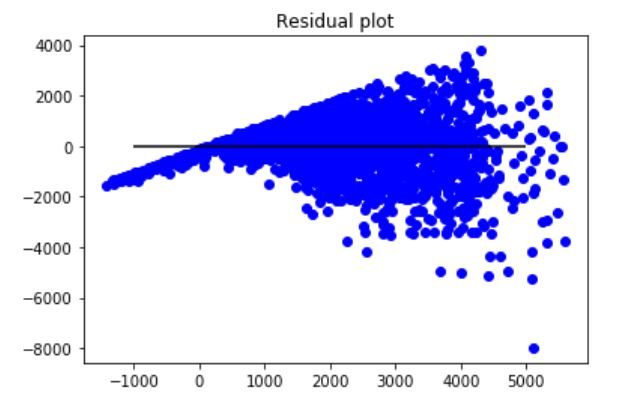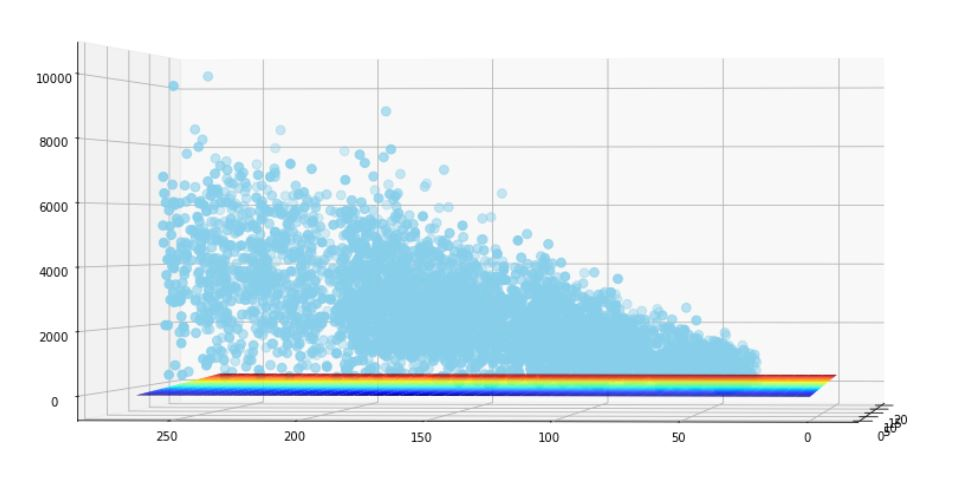# Heteroscedasticity (Heteroskedasticity)

Heteroscedasticity refers to the condition in which the variability of a variable is unequal across the range of values of a second variable that predicts it.

A scatterplot of these variables will often create a cone-like shape, as the scatter (or variability) of the dependent variable (DV) widens or narrows as the value of the independent variable (IV) increases.note: The inverse of heteroscedasticity is homoscedasticity, which indicates that a DV’s variability is equal across values of an IV.

You can also visualize that it will be difficult to find the hypothesis in the below scenario.Using the log transformation can be a good approach to correct for heteroskedasticity, but only if all your values are positive and the new model provides a reasonable interpretation. Heteroskedasticity does not bias our coefficient estimates; it only makes our standard errors incorrect. Hence, if you only care about the fit of the model, then heteroskedasticity doesn’t matter.

You can get a more efficient model (i.e., one with smaller standard errors) if you use weighted least squares.